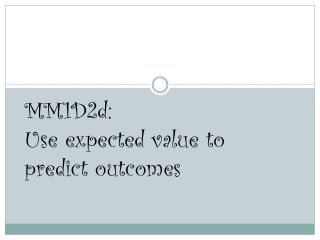DownloadDownload PresentationMM1D2d: Use expected value to predict outcomes

# MM1D2d: Use expected value to predict outcomes

Download Presentation## MM1D2d: Use expected value to predict outcomes

- - - - - - - - - - - - - - - - - - - - - - - - - - - E N D - - - - - - - - - - - - - - - - - - - - - - - - - - -
##### Presentation Transcript

1. MM1D2d:Use expected value to predict outcomes

2. Expected Value • The expected value is often referred to as the “long-term” average or mean . • This means that over the long term of doing an experiment over and over, you would expect this average. • To find the expected value or long term average, simply multiply each value of the random variable by its probability and add the products.

3. Example: • Suppose that the following game is played. A man rolls a die. If he rolls a 1, 3, or 5, he loses \$3, if he rolls a 4 or 6, he loses \$2, and if he rolls a 2, he wins \$12. What gains or losses should he expect on average? (What is his expected value?)

4. Step 1: • We must find the probabilities of each outcome. We can make a chart to help us see this.

5. Step 2: • Now, we multiply the probability for each outcome by the amount of money either gained or lost for that outcome. • Expected value of rolling a 1, 3, or 5: ________________ • Expected value of rolling a 4 or 6: ________________ • Expected value of rolling a 2: _______________

6. Step 3: • To find the expected value for the entire game (the answer), simply add up the expected value for each outcome. • Expected Value = • This means that the man playing this game is expected to lose an average of \$0.17 each game he plays.

7. Example: • Suppose that there is a raffle. Each ticket of the raffle cost \$1.00. There are 100 tickets sold for the raffle. The top prize is \$50.00; second prize receives \$10.00; and third prize receives \$1.00. What gains or losses should you expect on average? (What is the expected value?)

8. Step 1: • We must find the probabilities of each outcome. We can make a chart to help us see this.

9. Step 2: • Now, we multiply the probability for each outcome by the amount of money either gained or lost for that outcome. • Expected value of losing: -\$1(97/100)= -\$97/100= -\$0.97 • Expected value of 3rd: \$0 (1/100) = \$0 • Expected value of 2nd: \$10 (1/100) = \$10/100 = \$0.10 • Expected value of 1st: \$50 (1/100) = \$50/100 = \$0.50

10. Step 3: • To find the expected value for the raffle(the answer), simply add up the expected value for each outcome. • Expected Value = -\$0.97 +\$0.00 + \$0.10 + \$0.50 = -\$0.37 • This means that if you play this raffle you are expected to lose an average of \$0.37.

11. \$200 \$400 \$100 \$900 \$600 \$800 Spinner Example • What is the expected value of this spinner? • To find the expected value, add all the amount together. Then divide by the number of slices.

12. Step 1: • Add all the amounts on the spinner. • \$100+\$200+\$400+\$600+\$800+\$900 • \$3000 • Now divide by 6 slices; because there is a 1/6 probability of landing on any particular piece. • \$3000/6 • \$500 • The expected value of this spinner is \$500.

13. -\$200 \$400 -\$100 \$300 -\$600 \$800 Spinner Example • What is the expected value of this spinner? • To find the expected value, add all the amount together. Then divide by the number of slices.

14. Step 1: • Add all the amounts on the spinner together • -\$100-\$200+\$400-\$600+\$800+\$300 • \$600 • Now divide by 6 slices; because there is a 1/6 probability of landing on any particular piece. • \$600/6 • \$100 • The expected value of this spinner is \$100.

15. Extension • If you spin this same spinner 10 times, at the end what would you expect your outcome to be? How much money would you have? • \$100 x 10 = \$1000 • What is the probability of making at most \$400 on a single spin? • There are 5 pieces of the circle that are \$400 or less. • So probability is 5/6. • What is the probability of making at least \$300 on a single spin? • There are 3 pieces of the circle that are \$300 or more. • So probability is 3/6.

16. Extension • If you spin the spinner once and receive \$300 dollars, what is the probability of a sum of \$600 after the second spin? • \$600-\$300=\$300 • There is only one \$300 spot, so the probability is 1/6. • What is the probability of spinning the spinner twice and having a sum of at least \$400? • First need to make a chart of all the possible sums you can get from spinning the spinner twice.

17. Extension • What is the probability of spinning the spinner twice and having a sum of at least \$400? • No we have our table, count the number of spins that are \$400 or more. • 13 spins • Total possible spins = 36 • So probability is 13/36